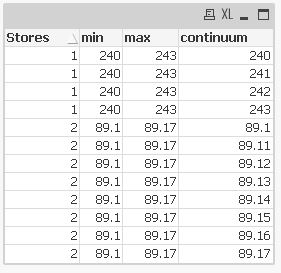# QlikView Scripting

Discussion Board for collaboration on QlikView Scripting.

HighlightedNot applicable

## Loop with customized decimal increment

Hi,

This is a sample data set.

Storesminmax
1240243
289.189.17

I want to create a continuum of values ranging from min to max for each store. So for Store 1, the values will be 240, 241, 242, 243. And for store 2, they will be 89.10, 89,11, 89.12,,....., 89.17.

I am writing a loop. However, the increments are in integers. How can I force the loop to increase by 0.01 for stores whose minimums are left in decimals? Here's my current code:

LET vNumRows = NoOfRows('C'); //// C is a previously created table

For Counter = 1 TO \$(vNumRows)

let vstoreLkup = Peek('store',\$(Counter)-1,C);

let vmin = Peek('min',\$(Counter)-1,C);

let vmax = Peek('max',\$(Counter)-1,C);

IF vmin=round(output) THEN  ////// output field is original. Some stores (like store 1) need to be rounded to get the min. Others (store 2)                                                             are left in  decimals

For vcontinuum = \$(vmin) to \$(vmax)

Concatenate(Data)

'\$(vstoreLkup)' as store,

'\$(vcontinuum)' as continuum,

'\$(vmin)' as  min,

'\$(vmax)' as max,

AutoGenerate 1;

next

ELSE

For vslider = \$(vmax) to \$(vmin)    /////Here is where I need to force the increments to be in decimal, I am thinking.

Concatenate(Data)

Concatenate(Data)

'\$(vstoreLkup)' as store,

'\$(vcontinuum)' as continuum,

'\$(vmin)' as  min,

'\$(vmax)' as max,

AutoGenerate 1;

next

ENDIF

next

Appreciate the help. Thank you.

Tags (2)
1 Solution

Accepted SolutionsMVP

## Re: Loop with customized decimal increment

Hi,

a simpler solution might be:```LOAD *,
min+If(Frac(min),0.01,1)*(IterNo()-1) as continuum
FROM [https://community.qlik.com/thread/194232] (html, codepage is 1252, embedded labels, table is @1)
While min+If(Frac(min),0.01,1)*(IterNo()-1)<=max;
```

hope this helps

regards

Marco

9 Replies
Contributor III

## Re: Loop with customized decimal increment

What about using a macro like;

Function getContinuum (min,max)

b=false

s=min & ";"

if( int(min)<>min or int(max)<>max) then

min = min * 100

max = max * 100

b=true

end if

do while min<max

min = min +1

if b then

s=s & min/100 & ";"

else

s=s & min & ";"

end if

loop

getContinuum=left(s,len(s)-1)

end function

Then in script:

*,

getContinuum(min,max) as continuum

Resident C;

Contributor III

## Re: Loop with customized decimal increment

or a little bit better:

Function getContinuum(min,max)

b=false

s=min & ";"

if( int(min)<>min or int(max)<>max) then

b=true

end if

do while min<max

if b then

min = min + 0.01

else

min = min + 1

end if

s= s & min & ";"

loop

getContinuum=left(s,len(s)-1)

end function

Valued Contributor III

## Re: Loop with customized decimal increment

Hi,

One approach could be using While in the load script:And here is the data model:I am also attaching the qvw.

Hope this helps.MVP

## Re: Loop with customized decimal increment

Hi,

a simpler solution might be:```LOAD *,
min+If(Frac(min),0.01,1)*(IterNo()-1) as continuum
FROM [https://community.qlik.com/thread/194232] (html, codepage is 1252, embedded labels, table is @1)
While min+If(Frac(min),0.01,1)*(IterNo()-1)<=max;
```

hope this helps

regards

MarcoNot applicable

## Re: Loop with customized decimal increment

Thank you everyone for your helpful responses. Using while solved the issue perfectly.

Regards,Not applicable

## Re: Loop with customized decimal increment

Hi Marco,

Thank you again for your suggestion on the while loop. As I mentioned earlier it did work. But upon further validation, I found that for some stores, the continuum does not reach the max value, even using the same logic. I have attached my qvw. Max from both stores is 0.54. But for store #1, continuum does not reach the max value. It stops at 0.55. Store #2 works as it should. In this case, lower is better. So I am subtracting .01 from the min value each time until it reaches the max.

It seems that the min value for store 1 (ceil(0.70395633,.01)= .71) is the problem. Because if you change the min to max and do while loop with .01 increment, for store 1, continuum goes up to only 0.7. This code is commented in the qvw.

Also, if you look at the continuum values, sometimes it is creating two sets of continuum values over the same range for each of the stores.

Any help or explanation would be greatly appreciated. I am totally stumped as to why this would not be working. Thank you in advance.

Contributor III

## Re: Loop with customized decimal increment

check this outstore,min,max,

IterNo() as i,
0.01*(
IterNo()-1) as step,

min + 0.01*(IterNo()-1) as currentvalue
WHILE min*100 + IterNo() - 1  <= max * 100
;

store,

floor(target, .01)   as min,

ceil(score, .01)     as max
;

INLINE
[
store, score, target

1, 0.70395633, 0.5479

2, 0.66028157, 0.5479

]
;Not applicable

## Re: Loop with customized decimal increment

Thanks Boris. Tried it on Friday. It seems to work. I will validate further today. Hopefully, it will work perfectly!

Contributor III

## Re: Loop with customized decimal increment

Hi Marco,

Code is looks prefect to generate decimal loop but it is not working when we go to Number 0.9 i.e

if My Min=19.9 then as per code its is taking as 19.1 can it take as 19.10

Regards,

Karim Khan